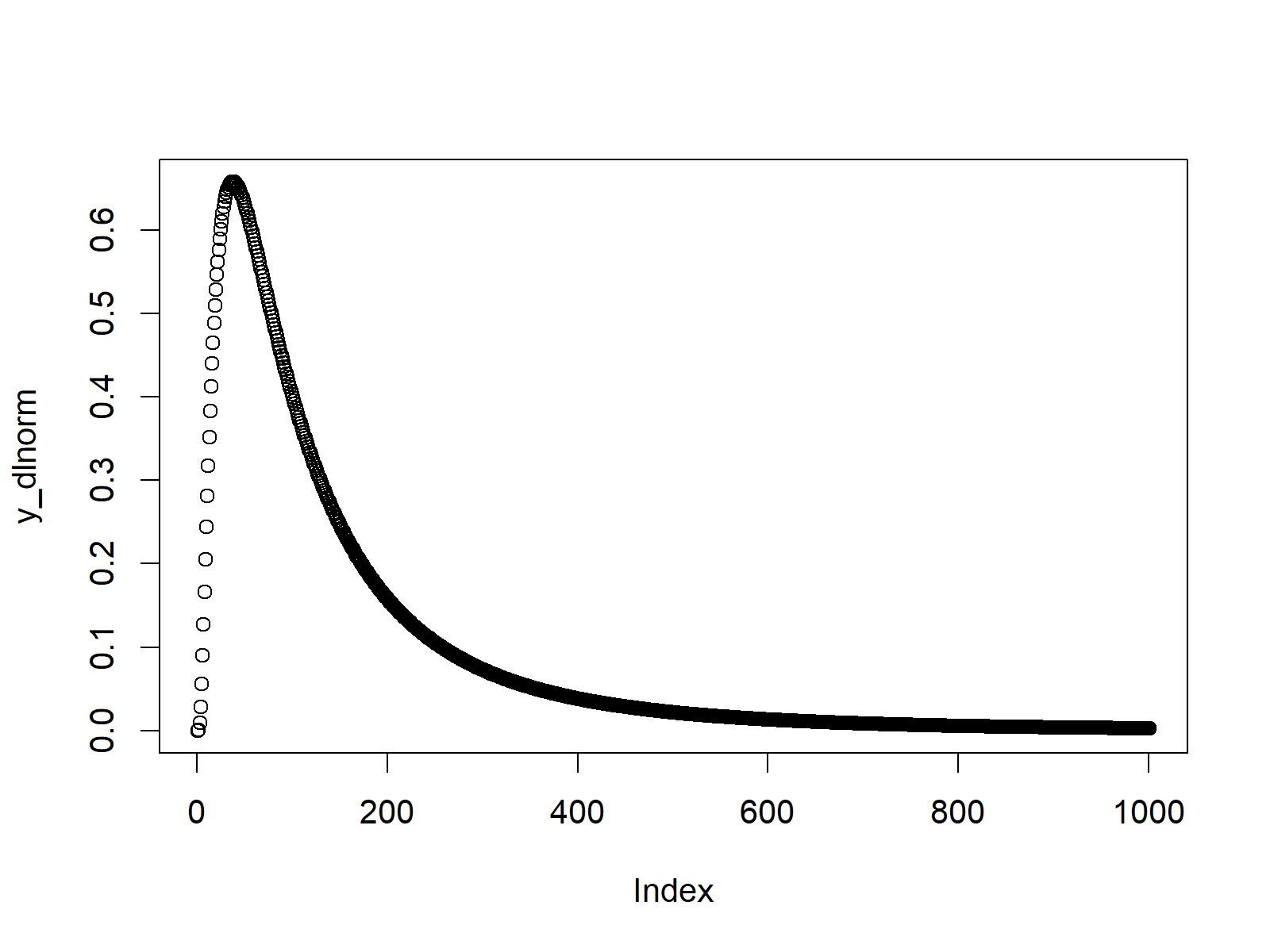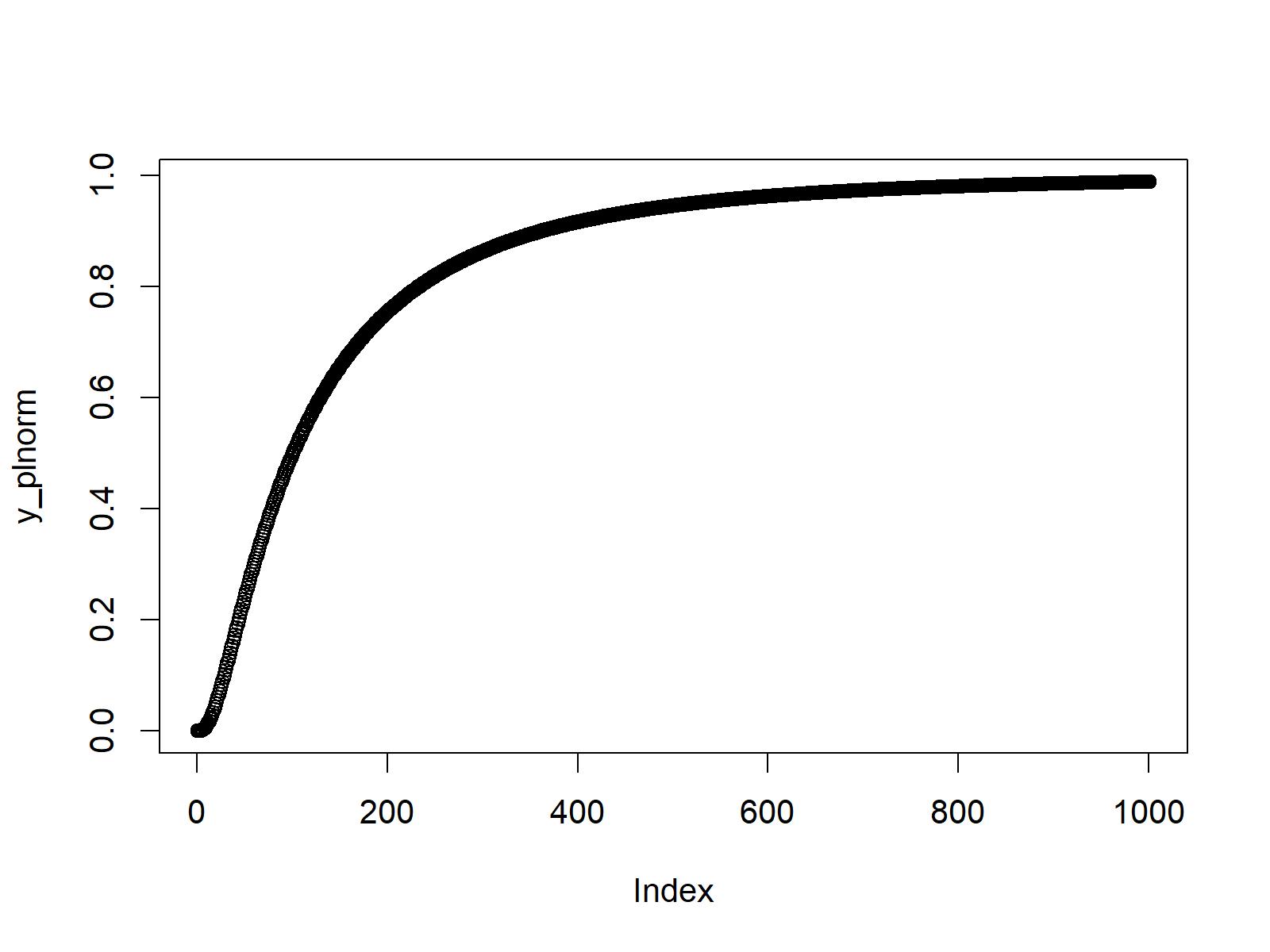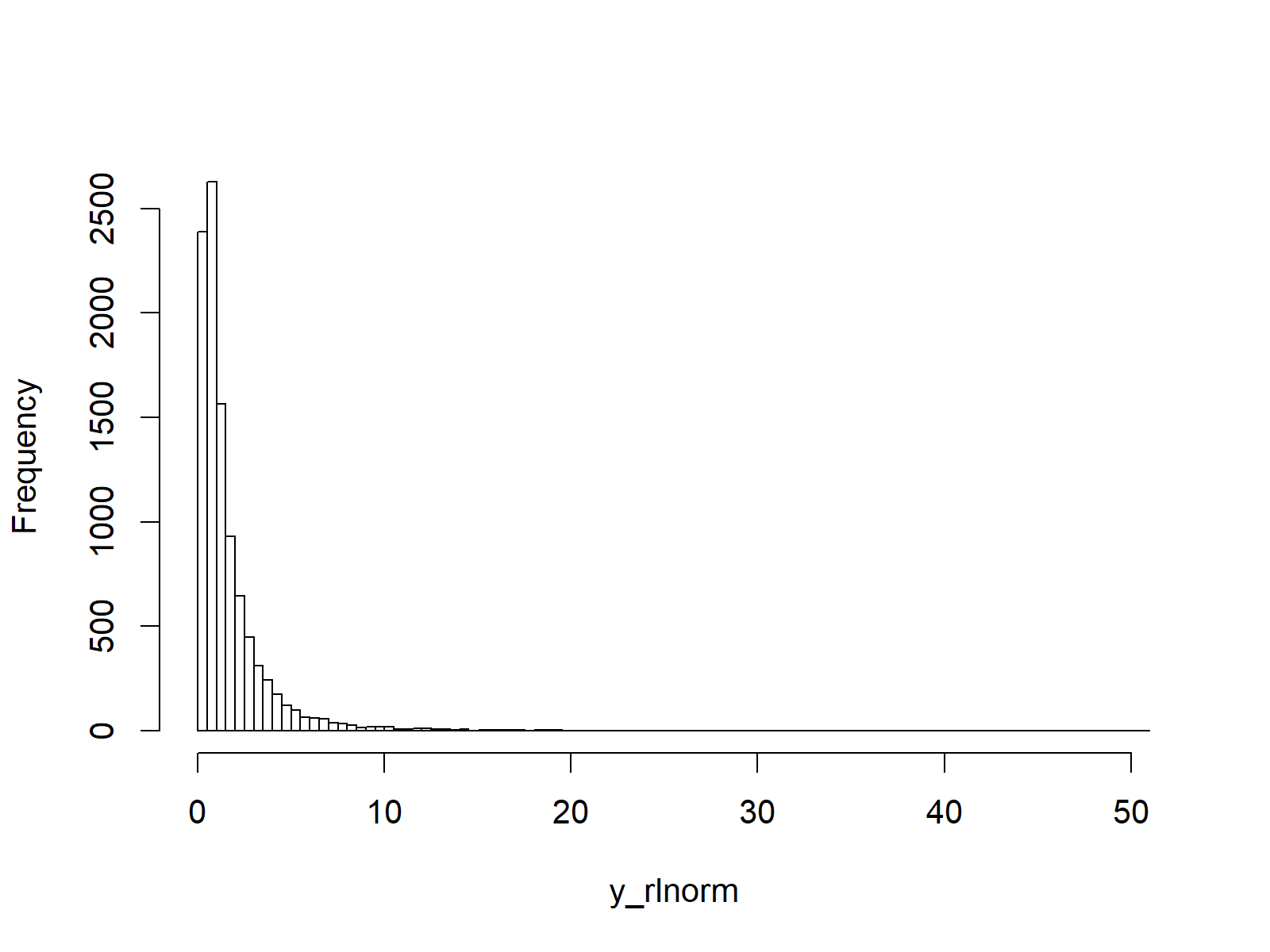# Log Normal Distribution in R (4 Examples) | dlnorm, plnorm, qlnorm & rlnorm Functions

This tutorial shows how to apply the log normal functions in R.

The page consists of this content:

Let’s dig in!

## Example 1: Log Normal Probability Density Function (dlnorm Function)

In the first example, I’ll show you how the log normal density looks like. First, we need to create a sequence of quantile values that we can use as input for the dlnorm R function.

`x_dlnorm <- seq(0, 10, by = 0.01)                       # Specify x-values for dlnorm function`

Now, we can apply the dlnorm function as follows:

`y_dlnorm <- dlnorm(x_dlnorm)                            # Apply dlnorm function`

The previous R code stored the output of the dlnorm function in the data object y_dlnorm. We can now use the plot function to draw a graphic, representing the probability density function (PDF) of the log normal distribution:

`plot(y_dlnorm)                                          # Plot dlnorm values`Figure 1: PDF of Log Normal Distribution.

## Example 2: Log Normal Cumulative Distribution Function (plnorm Function)

Example 2 shows how to draw the cumulative distribution function (CDF) of the log normal distribution. Again, we need to create a vector of quantiles:

`x_plnorm <- seq(0, 10, by = 0.01)                       # Specify x-values for plnorm function`

And then, we need to insert this vector into the plnorm command:

`y_plnorm <- plnorm(x_plnorm)                            # Apply plnorm function`

We can draw the cumulative distribution function as follows:

`plot(y_plnorm)                                          # Plot plnorm values`Figure 2: CDF of Log Normal Distribution.

## Example 3: Log Normal Quantile Function (qlnorm Function)

In Example 3, we’ll create the quantile function of the log normal distribution. As a first step, we have to create a sequence of probabilities (i.e. values between 0 and 1):

`x_qlnorm <- seq(0, 1, by = 0.01)                        # Specify x-values for qlnorm function`

Then, we can apply the qlnorm function to this sequence:

`y_qlnorm <- qlnorm(x_qlnorm)                            # Apply qlnorm function`

Finally, we can draw our quantile function plot of the log normal distribution:

`plot(y_qlnorm)                                          # Plot qlnorm values`Figure 3: Quantile Function of Log Normal Distribution.

## Example 4: Generating Random Numbers (rlnorm Function)

In the last example of this R tutorial, I’ll explain how to draw random numbers according to the distribution of the log normal density. First, we need to set a seed and specify the amount of random numbers that we want to simulate:

```set.seed(91929)                                         # Set seed for reproducibility
N <- 10000                                              # Specify sample size```

Then, we can apply the rlnorm function in order to generate N random numbers:

```y_rlnorm <- rlnorm(N)                                   # Draw N log normally distributed values
y_rlnorm                                                # Print values to RStudio console
# 0.88082919  0.71130233  1.55750385  0.74597213  1.12296291  1.73100566  0.72801951  1.25833372  2.09056650...```

As you can see based on the previous RStudio console output, our random numbers are stored in the data object y_rlnorm. We can draw a histogram showing the distribution of these random numbers as shown below:

```hist(y_rlnorm,                                          # Plot of randomly drawn log normal density
breaks = 100,
main = "")```Figure 4: Random Numbers Distributed as Log Normal Distribution.

As you can see: The random numbers are distributed as the log normal distribution. Looks fine!

## Video & Further Resources

In case you need more info on the R programming syntax of this page, I can recommend to watch the following video of my YouTube channel. I show the examples of this tutorial in the video:

You might also read the other articles on probability distributions and the simulation of random numbers in R:

In addition to the video, I can recommend to read the other articles on my website:

This tutorial illustrated how to use the log normal functions in R programming. Please tell me about it in the comments section, if you have further questions.

Subscribe to the Statistics Globe Newsletter